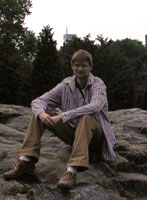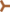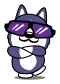Who?

I am a Senior Lecturer at the Department of Mathematics at Stockholm University, and co-founder of dogl.

Education-wise, I am interested in making concepts tangible and hands-on.

Research-wise, my main interests lie in a mixture of harmonic analysis, probability, combinatorics, and number theory.

CV

Google Scholar provides some interesting links to papers and articles surrounding my research.Contact details
You can usually reach me at olof.sisask[AT-tecken]math.su.se.Dogl Calculus

Together with a fantastic team, I'm trying to make it easier for Calculus students to engage with solving problems. Through a mobile app. While still having it be pedagogically sound! Check out Dogl Calculus for our progress so far.Public engagement
Have fun and understand stuff with interactive tools!
The text is in Swedish, but the ideas universal. Unfortunately these are not yet fully self-contained, and might not work terribly well on mobile phones, but they will hopefully make sense to you if you attended one of my public lectures or the teacher development day Kleinerdagen at the Royal Swedish Academy of Sciences (KVA). If you want any of the other materials we used, please email me!

Want to study the emergence of a giant puddle in the random raindrop model? Here's a visualisation. (Puddles merge according to their barycentres.)Teaching

For my notes in other subjects, please see the respective course webpages.Papers and articles
• The Kelley–Meka bounds for sets free of three-term arithmetic progressions
With Thomas Bloom. Preprint.
• Breaking the logarithmic barrier in Roth's theorem on arithmetic progressions
With Thomas Bloom. Submitted.
• Logarithmic bounds for Roth's theorem via almost-periodicity.
With Thomas Bloom. Discrete Analysis 2019:4 20pp.
• Convolutions of sets with bounded VC-dimension are uniformly continuous.
Discrete Analysis 2021:1 25pp.
• Roth's theorem for four variables and additive structures in sums of sparse sets.
With Tomasz Schoen. Forum of Mathematics, Sigma 4 (2016) e5 (28 pages).
• Convergence results for systems of linear forms on cyclic groups, and periodic nilsequences.
With Pablo Candela. SIAM J. Discrete Math. 28 (2) (2014), 786–810.
• Arithmetic progressions in sumsets and Lp-almost-periodicity.
With Ernie Croot and Izabella Laba. Combin. Probab. Comput. 22 (3) (2013), 351–365.
• A removal lemma for linear configurations in subsets of the circle.
With Pablo Candela. Proc. Edinburgh Math. Soc. 56 (3) (2013), 657–666.
• On the asymptotic maximal density of a set avoiding solutions to linear equations modulo a prime.
With Pablo Candela. Acta Math. Hungar. 132 (3) (2011), 223–243.
What is the largest density of a subset of Z/pZ that does not contain a solution to either of the equations x1 + x2 = x3, x1 + 2x2 = 4x3 + 5x4? This paper shows that if p is large then the answer to such questions is essentially given by an analogous quantity in R/Z, answering a Z/pZ-version of a question of Ruzsa about subsets of [N].
• A probabilistic technique for finding almost-periods of convolutions.
With Ernie Croot. Geom. Funct. Anal. 20 (2010), 1367–1396.
• On the maximal number of three-term arithmetic progressions in subsets of Z/pZ.
With Ben Green. Bull. London Math. Soc. 40 (2008), 945–955.
• A new proof of Roth's theorem on arithmetic progressions.
With Ernie Croot. Proc. Amer. Math. Soc. 137 (2009), 805–809.
• A family of large density, large diameter sum-free sets in Z/pZ.
Work of Deshouillers, Freiman and Lev has shown that large sum-free subsets of Z/pZ are necessarily somewhat structured, in the sense that they have a dilate contained in a short interval. In particular, this is known to hold for sets of density at least 0.318. In this note we construct a family of sum-free sets of density 0.25 that do not have a dilate contained in a short interval.
(still needs polishing) | abstract
• Freiman isomorphisms between characters and linear limits of groups.
We prove that the minimum number of 3APs in a subset of Z/pZ of density delta, divided by p2, is the same as the minimum amount of 3APs in a subset of the R/Z of density delta, up to o(1) errors as p tends to infinity. In fact, our results are rather more general than this, dealing with the general question of moving between linear equation counts over any compact abelian groups. E-mail me if interested!
(still needs polishing) | briefNotes
• Bourgain's proof of the existence of long arithmetic progressions in A+B.
These are some notes I wrote while trying to understand Bourgain's proof of the existence of long arithmetic progressions in sumsets A+B. I found it easiest to think of Bourgain's work as establishing an Lp-almost-periodicity result for convolutions of functions.
• An additive combinatorial take on Zeta constants.
This is a short note demonstrating how one may interpret the values of Zeta(2), Zeta(4) and related sums in an additive combinatorial fashion. The basic idea is that one can view these values as representing the number of solutions to some linear equation in a simple subset of Z/pZ. What about Zeta(3)? Read the note and try it yourself!